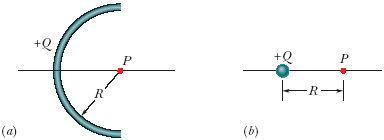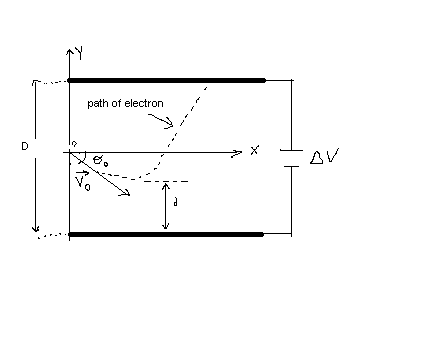Ask question

# Find the radius, if s=38,000cm theta=45.3^circ Use the arc length formula.# Find the radius, if s=38,000cm theta=45.3^circ Use the arc length formula.

Question
Analytic geometryasked 2020-12-28
Find the radius, if
s=38,000cm
$$\displaystyle\theta={45.3}^{\circ}$$
Use the arc length formula.

## Answers (1)2020-12-29
We're given:
s=38,000cm
$$\displaystyle\theta={45.3}^{\circ}$$
First, convert $$\displaystyle\theta$$ from degrees to radians:
$$\displaystyle\theta={45.3}^{\circ}\times\frac{{\pi}}{{{180}^{\circ}}}$$
$$\displaystyle=\frac{{{45.3}\pi}}{{180}}$$
The arc length formula:
$$\displaystyle{s}={r}\theta$$
Where r=radius. Since that's what we need to find, isolate it:
$$\displaystyle{r}=\frac{{s}}{\theta}$$
Substitute s and $$\displaystyle\theta$$:
$$\displaystyle{r}=\frac{{{38},{000}{\left({180}\right)}}}{{{45.3}\pi}}$$
$$\displaystyle{r}\approx{48062.7}$$ cm

### Relevant Questionsasked 2021-04-15
A car initially traveling eastward turns north by traveling in a circular path at uniform speed as in the figure below. The length of the arc ABC is 235 m, and the car completes the turn in 33.0 s. (Enter only the answers in the input boxes separately given.)
(a) What is the acceleration when the car is at B located at an angle of 35.0°? Express your answer in terms of the unit vectors $$\displaystyle\hat{{{i}}}$$ and $$\displaystyle\hat{{{j}}}$$.
1. (Enter in box 1) $$\displaystyle\frac{{m}}{{s}^{{2}}}\hat{{{i}}}+{\left({E}{n}{t}{e}{r}\in{b}\otimes{2}\right)}{P}{S}{K}\frac{{m}}{{s}^{{2}}}\hat{{{j}}}$$
(b) Determine the car's average speed.
3. ( Enter in box 3) m/s
(c) Determine its average acceleration during the 33.0-s interval.
4. ( Enter in box 4) $$\displaystyle\frac{{m}}{{s}^{{2}}}\hat{{{i}}}+$$
5. ( Enter in box 5) $$\displaystyle\frac{{m}}{{s}^{{2}}}\hat{{{j}}}$$asked 2020-11-03
Given:
$$\displaystyle\theta={240}^{\circ}$$
Find the value of $$\displaystyle\theta$$ in radians (in terms of $$\displaystyle\pi$$)asked 2021-03-09

Given $$\displaystyle{r}′{\left({t}\right)}=⟨{\sec{{2}}}{t},−{\sin{{t}}}⟩$$, find the arc length of the curve r(t) on the interval $$[-\pi/3]$$asked 2021-02-03
A circle is inscribed in a right triangle. The length of the radius of the circle is 6 cm, and the length of the hypotenuse is 29 cm. Find the lengths of the two segments of the hypotenuse that are determined by the point of tangency.asked 2020-11-08
Find the six function values of $$\displaystyle{8}^{\circ}$$ in terms of p, q and r, if
$$\displaystyle{{\sin{{82}}}^{\circ}=}{p}$$
$$\displaystyle{{\cos{{82}}}^{\circ}=}{q}$$
$$\displaystyle{{\tan{{82}}}^{\circ}=}{r}$$asked 2021-03-03
a)b)Figure shows a nonconducting rod with a uniformly distributed charge +Q. The rod forms a 10/22 of circle with radius R and produces an electric field of magnitude Earc at its center of curvature P. If the arc is collapsed to a point at distance R from P, by what factor is the magnitude of the electric field at P multiplied?asked 2021-05-12
An electron is fired at a speed of $$\displaystyle{v}_{{0}}={5.6}\times{10}^{{6}}$$ m/s and at an angle of $$\displaystyle\theta_{{0}}=–{45}^{\circ}$$ between two parallel conductingplates that are D=2.0 mm apart, as in Figure. Ifthe potential difference between the plates is $$\displaystyle\triangle{V}={100}\ {V}$$, determine (a) how close d the electron will get to the bottom plate and (b) where the electron will strike the top plate.asked 2021-01-10

$$\displaystyle{\cos{{2}}}\theta=\frac{{28}}{{53}}$$
Find $$\displaystyle{\sin{\theta}}$$, if
$$\displaystyle{0}^{\circ}{<}\theta{<}{90}^{\circ}$$asked 2021-01-08
Multiple Choice For Exercises 1−6, choose the correct letter. For Exercises 1 and 2 , use the diagram at the right.
The figure at the right is a Ferris wheel in the shape of a regular octagon with spokes extending from the center to each vertex and an apothem drawn. What is m∠1? A. 22.5 B. 45 C. 60 D. 67.5asked 2021-04-13
As depicted in the applet, Albertine finds herself in a very odd contraption. She sits in a reclining chair, in front of a large, compressed spring. The spring is compressed 5.00 m from its equilibrium position, and a glass sits 19.8m from her outstretched foot.
a)Assuming that Albertine's mass is 60.0kg , what is $$\displaystyle\mu_{{k}}$$, the coefficient of kinetic friction between the chair and the waxed floor? Use $$\displaystyle{g}={9.80}\frac{{m}}{{s}^{{2}}}$$ for the magnitude of the acceleration due to gravity. Assume that the value of k found in Part A has three significant figures. Note that if you did not assume that k has three significant figures, it would be impossible to get three significant figures for $$\displaystyle\mu_{{k}}$$, since the length scale along the bottom of the applet does not allow you to measure distances to that accuracy with different values of k.
...